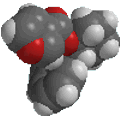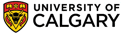Useful Concepts### Optical Purity and all that....

Molecules with chirality centers cause the rotation of plane polarised light and are said to be "optical active" (hence the term optical isomers).
Enantiomeric molecules rotate the plane in opposite directions but with the same magnitude.
This provides a means of measuring the "optical purity" or "enantiomeric excess (ee)" of a sample of a mixture of enantiomers.
These two terms mean the same thing : How much more of one enantiomer is there than the other ?

Specific rotation is a physical property like boiling point and can be looked up in references. It is defined according to the following equation based on the experimental measurements:

Specific rotation [α]D = αobs / c l

where "αobs" is the experimentally observed rotation, "c" is the concentration in g/ml and "l" is the pathlength of the cell used expressed in dm (10 cm).
The most important factor is that two enantiomers will have the same magnitude specific rotations but in opposite directions.

For example: (S)-bromobutane has a specific rotation of +23.1o , therefore, (R)-bromobutane has a specific rotation of -23.1o

As a consequnce of this, a 50:50 mixture of the two enantiomers will not rotate plane polarised light because the effects of the two enantiomers cancel each other out, molecule for molecule. This type of mixture is called a racemate or a racemic mixture. The specific rotation of a racemic mixture is zero.

The optical purity of a mixture of enantiomers is given by:

% Optical purity of sample = 100 * (specific rotation of sample) / (specific rotation of a pure enantiomer)

Based on the above example data for the bromobutanes:

Optical purity of a racemic mixture = 100 * (0o) / (+23.1o) = 0%     i.e. there is no one enantiomer present in excess.

Another way to express optical purity is as the "enantiomeric excess" or ee:

ee % = 100 * ( [ R ] - [ S ] ) / ( [ R ] + [ S ] )

where [-] indicates the concentration of the species. Repeating the above example (to show the identity) in a racemic mixture [ R ] = [ S ], therefore,

ee% = 100 * 0 / ( [ R ] + [ S ] ) = 0%

or, if we use the % as concentrations, then [ R ] = [ S ] = 50 % we get,

ee% = 100 * (50 - 50 )/ (50 + 50 ) = 0 %

So, what about for a pure enantiomer, say 100% R ?

Optical purity % = 100 * (-23.1o / -23.1o) = 100 %, or, ee% = 100 * (100% - 0%) / (100% + 0%) = 100%

So, finally, what about another mixture of the 2-bromobutanes of measured specific rotation = -9.2o ?
Well the fact that the sign is negative tells us that in this case the R enantiomer is the dominant one.

Optical purity % = 100 * (- 9.2o / - 23.1o) = 40 % ie there is a 40% excess of R over S.

This corresponds to a mixture of 70% R and 30% S. How do you get this quickly ?

Well, if there is a 40% excess of R, then the 60% leftover must be equal amounts of both R and S ie. 30% of each.  So the total amount of R is 30% + 40% excess = 70%.

You should also be able to work out the specific rotation of a mixture given the % composition. Try it out for the above example.

As a further example for the same system, what would the optical purity be is the measured specific rotation were +18.4o ? Now the enantiomer in excess must be the S.

Optical purity% = 100 * (+18.4o / +23.1o) = 80% corresponding to a 90% S : 10% R mixture.

OK ?© Dr. Ian Hunt, Department of Chemistry